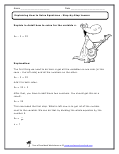Home > Topics >

## Expressions and Equations WorksheetsA super common question is the difference between expressions and equations. Expressions can be evaluated, but never truly have a single solution. The evaluation of expressions change based on the variables involved. Equations can be solved and often the make two expressions equal.

Expression: 8x + 12 (Different solutions based on the value of x)

Equation: 8x + 12 = 52 (There is only 1 value for x)

Basic Operations and Generating Equivalent Expressions

Completing the Square in a Quadratic Expression

Complicated Expressions

Creating and Identifying Equivalent Expressions

Creating Equations and Inequalities

Creating Equations with Two or More Variables

Evaluating Numerical Expressions with Exponents

Evaluating Written Expressions

Explaining How to Solve Equations

Expressions That Use Math Terms

Expressions Used In Word Problems

Finding Points of Intersection for Complex Equations

Graphing Equations

Graphing Linear Inequalities as a Half-Plane

Graphing Proportional Relationships

Inequality Constraint or Condition Word Problems

Interpret the Context of Expressions

Linear Equations in One Variable

Powers of Ten and Scientific Notation

Properties of Integer Exponents

Using Tables and Data Charts With Expressions

Using Variables to Represent Numbers

Using Variables to Represent Two Quantities

Rearranging and Understanding Formulas

Rewriting Expressions - Middle School Level

Rewriting Expressions - High School Level

Real Life Middle School Math Word Problems

Scientific Notation Word Problems

Simultaneous Linear Equations

Solve One-Variable Equations and Inequalities

Solve Rational and Radical Equations

Solve Real-world Mathematical Problems With Expressions

Solving Equations and Inequalities

Solving Linear Equations and Inequalities in One Variable

Solving Linear Expressions

Solving Simultaneous Equations (Linear and Quadratics)

Solving Systems of Equations

Solving Systems of Linear Equations by Graphing

Solving Systems Word Problems

Square and Cube Roots

Two Linear Equations in Two Variables

Using Graphs of Equations

Word Problems Leading to Equations

Word Problems Leading to Inequalities

Word Problems That Require Equations or Inequalities

Writing Expression for Geometric Sequences